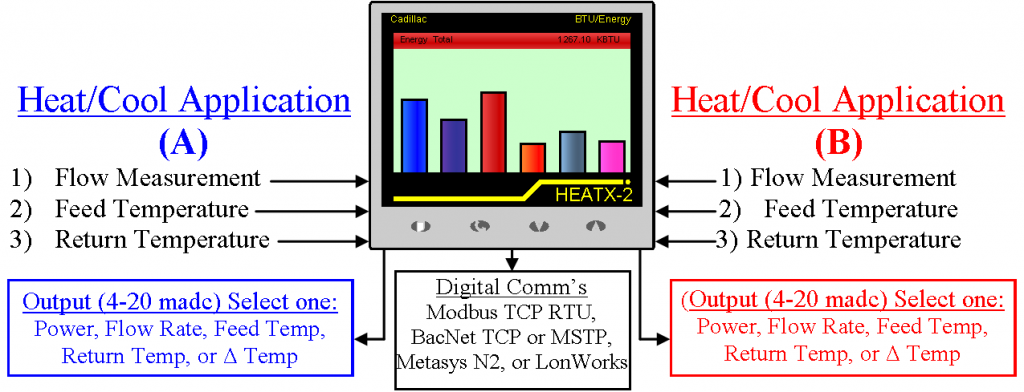# Deriving The Formula to Measure The Energy Content of Liquid FlowWhen considering energy usage in a typical heating or cooling water system a calculation must be made to determine the difference between what was supplied to the source of heating or cooling versus what was returned. This calculation of the supply versus the return energy difference is called the Btu meter formula

In the case of providing heat energy, the liquid will be supplied from a heat source (boiler, heat exchanger, etc,) at a certain volumetric rate and at a certain temperature to a heat sink (radiator, fan coil unit, heat exchanger, etc) and then return back to the heat source at the same volumetric rate, but at a lower temperature. With this in mind the only variable that changes during this energy exchange is the temperature of the liquid media. So, if we are able to measure the volumetric flow rate in either the feed or return lines, and at the same time measure both temperatures in the feed and return lines we have the basis for deriving the Btu meter formula.

At this point the Btu Meter Formula will consist of the following variable measurements:

1) Volumetric flow rate = Volume of liquid/time – Typically Gallons/Minute (GPM) or Liters/Minute (L/m)

2) Temperature of Feed = Temperature units – Typically Fahrenheit (°F) or Celsius (C°)

3) Temperature of Return = Temperature units – Typically Fahrenheit (°F) or Celsius (C°)

Refining further the Btu Meter formula = Volumetric flow rate *∆T (Feed Temp – Return Temp)

However considering the units of measure, the Btu Meter formula would only calculate = Volume (gal) *∆T(°F)/Time (min). So, in order to calculate Btu’s/time we must convert the variables being measured into units to derive energy usage. To do so we must look at the desired result of the Energy units we would like to calculate. In this case we would like to calculate power since the flow is dynamic or has a time component. Typically heating power for liquid flow would be Btu/Hr.

In order to convert the measured variables to Power or Btu/Hr from Volumetric flow rate *∆T (Feed Temp – Return Temp) the Btu Meter formula evolves to the following:

P= V x ρ x (hTV –hTR)

Where  P = Power (BTU/Hr)

V = Volumetric flow rate (Gal/Hr)

ρ = Density (Lb/Gal)

hTV = Specific Enthalpy (BTU/Lb) – Feed Temperature

hTR = Specific Enthalpy (BTU/Lb) – Return Temperature

To convert Volumetric flow to from Gal/min to Gal/Hr, we would take Gal/min and multiply 60 minutes = (gal/min)*(60 min/hr) = Gal/Hr.

To convert Gallons to Lbs, we need to look at the density of water as a function of temperature.  At room temperature or approximately 70°F a gallon of water would weigh 8.33 Lbs. At 130F a gallon of water would weigh 8.205 Lbs.

To convert the ∆T (Feed Temp- Return Temp) of the liquid (water) to (Btu/Lb) we must look at the enthalpy of water as a function of temperature. While the enthalpy values vary from those of temperature the difference or ∆T are essentially the same. See the example below

Feed Temp = 130°F                          Feed Temp @ 130°F = hTV = 97.99 Btu/Lb

Return Temp = 120°F                      Feed Temp @ 120°F = hTV = 88.00 Btu/Lb

∆T = (130°F – 120°F) = 10°F            (hTV –hTR) = (97.99Btu/Lb – 88.0 Btu/Lb) = 9.99 Btu/Lb

At this point the Btu meter formula is complete with the three dynamic measurement variables of Volumetric flow rate, feed temperature and return temperature as shown below:

Measured variables with flow measurement taken in the feed line:

Flow rate = 60 Gal/Min

Feed temp = 130F

Return Temp = 120F

Plugging these into the Btu meter formula we have the following:

Power (Btu/hr) = (60 Gal/Min)*(60 Min/Hr)*(8.205 Lbs/Gal)*(97.99 Btu/Lb – 88.0 Btu Lb)

Power = 295,085 Btu/Hr

For a quick check of the Btu meter formula, you can use the following short cut or “rule of thumb”:

Power (Btu/Hr) = Flow Rate*∆T*500

Flow rate = Gallons/Min

∆T = °F

500 = 60 (min/hr)*8.333 (Lbs/Gal)

Applied to above example:

Flow rate = 60 Gal/Min

Feed temp = 130F

Return Temp = 120F

Power (Btu/Hr) = (60)(10)(500)

Power = 300,000 Btu/Hr